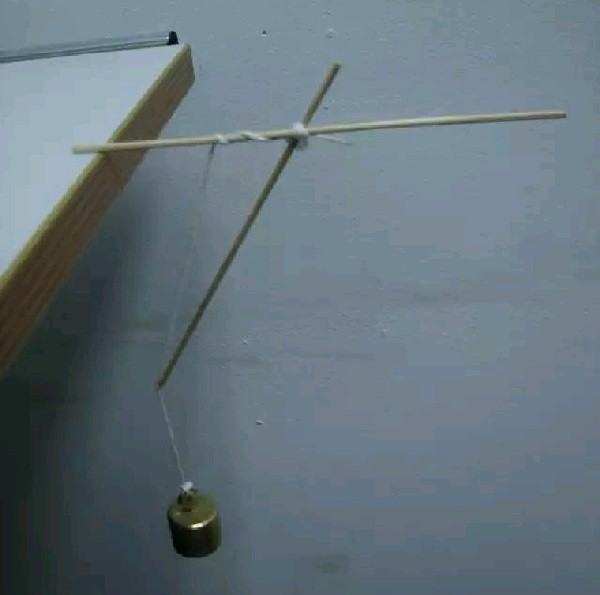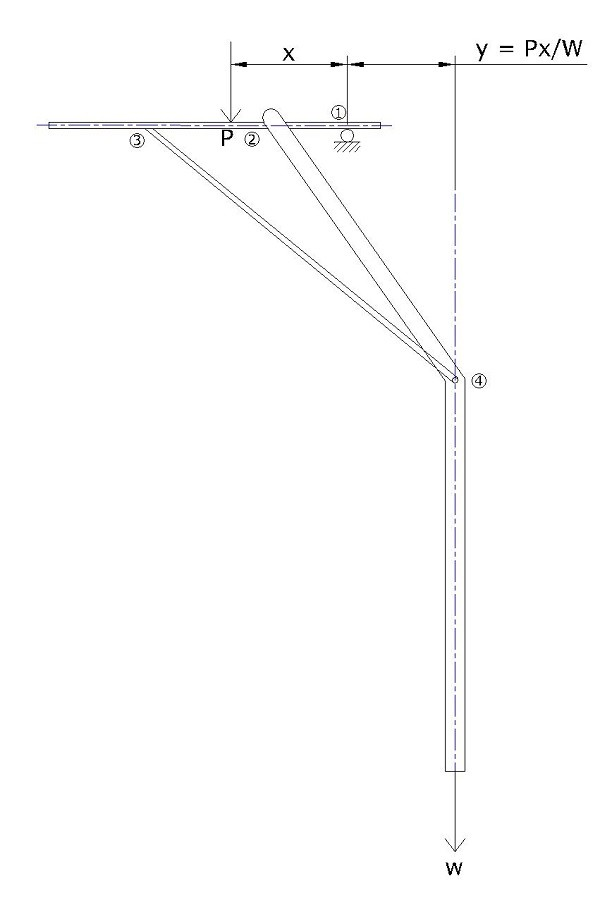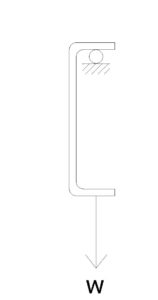Lorem ipsum dolor sit amet, consectetuer adipis cing elit. Aenean commodo ligula eget dolor. Aenean massa. Cum sociis theme natoque

TOP## A contrivance claimed to be self-balancing## A contrivance claimed to be self-balancing

Explaining a popular viral video with a bottle magically hanging from the edge of a table.

While we write this post in June 2020, during COVID times, there is a video that is widely circulated on social media, showing a bottle of water magically defying gravity by hanging from a cantilever without counterweight. This is the video:

Let us try to explain this phenomenon, in an engineering sense.In the video, weight W hangs on a two-fold rope, from a horizontal beam 1-2-3. The beam just bears on the table, that is similar to a roller support: no horizontal restraints or fixity. A strut 3-4 is claimed to provide an additional support.Let us assume that the strutting causes the rope to kink at Point 4. That is, the strut at an angle of B with vertical causes the rope to make an angle of A (hopefully, zero) with vertical. On the beam, the rope (Point 2) is at a distance a from the edge of the table (Point 1) and strut (Point 1) is at a distance b. The overall centre of mass of the hanging weight W is considered to be at a distance of x from the edge of the table.

As there is no other external support, Reaction R = W

Equilibrium of forces in beam 1-2-3: C. Cos (B) – T. Cos (A) = W

Resolving forces at Joint 3: Tension in the beam (2 to 3) = C. Sin(B)

Resolving forces at Joint 2: Tension in the beam (2 to 3) = T. Sin(A)

1. Sin(B) = T. Sin(A)

Note: if A = 0, then C = 0. That is, the rope is always kinked, it cannot be vertical when strutted.

Equilibrium of Moment about Point 1: Cb. Cos(B) = Ta. Cos(A)

Tb. Sin(A). Tan(B) = Ta. Cos(A)

a/b = Tan(A)/Tan(B)

But, Tan(A)/Tan(B) = (a-x)/(b-x). Either x = 0 or a = b

Thus, x = 0 is true because a = b is a trivial solution which is true only for W = 0

In other words, the strut should tuck the weight W under the table so that centre of mass is directly below the edge of the table.

1. Sin(A) Cos(B) – T. Cos(A) Sin (B) = W Sin(B)

T = W Sin(B) / Sin (B-A)

C = W Sin(A) / Sin (B-A)

Interestingly, tie beam 3-2 and strut 3-4 can act as a cantilever system to carry additional loads by pushing the weight, further below the table.Also, the arrangement is possible only when the connections at 2, 3 and 4 are correctly arranged. Point 3, requires a tongue and groove arrangement. Point 4 requires a similar groove or is probably held in place by friction.If all the joints were rigid enough to prevent all opening up, this is as good as following arrangement: